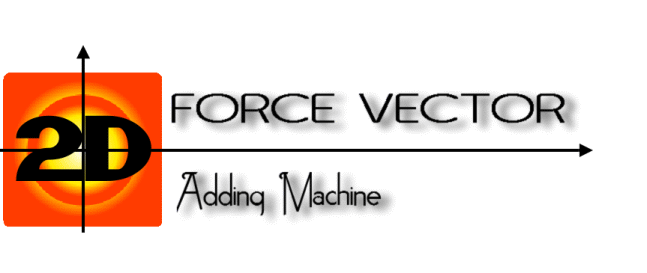# 2-D Force Vector Adding MachineThe 2-D Force Vector Adding Machine lets you visualize the sum of two force vectors, F1 and F2, using the component method of addition. You can vary the size (magnitude) and direction of F1 and F2.

The sum of F1 and F2 is named FT, for 'Force Total'. So:

FT = F1 + F2

Use the number adjusters to change the size and direction of F1 and F2.

### The y-component of FT is in an even lighter blue.

The angles A1, A2, and AT respectively describing the direction of F1, F2, and FT are in degrees and are to be considered in standard position.

All numbers are rounded to two decimal points. Numbers such as '8.00' will be shown as '8'.

The checkboxes control the visibility of their related part of the diagrams. For example, if you uncheck the box next to F1x, then the F1x component vector will no longer be drawn in the diagrams.

Custom Search